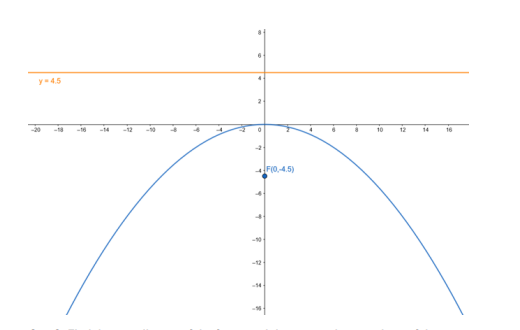# Find the coordinates of the focus and the vertex, the equations of the`
Question:

Find the coordinates of the focus and the vertex, the equations of the directrix and the axis, and length of the latus rectum of the parabola :

$x^{2}=-18 y$

Solution:

Given equation : $x^{2}=-18 y$

Comparing given equation with parabola having equation,

$x^{2}=-4 a y$

$4 a=18$

$\cdot a=\frac{9}{2}$

Focus: $F(0,-a)=F\left(0,-\frac{9}{2}\right)$

Vertex: $A(0,0)=A(0,0)$

Equation of the directrix: $y-a=0$

$y-\frac{9}{2}=0$

$y=\frac{9}{2}$

Lenth of latusrectum : $4 \mathrm{a}=18$